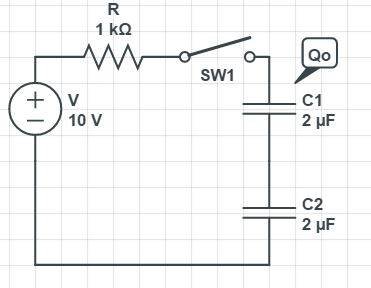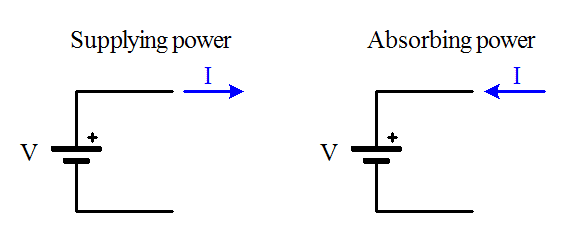# Find the total work in a circuit

• Engineering

## Homework Statement

This is a problem from some textbook a friend emailed me:The text goes like this:
When the switch is open the charge on ##C1## equals ##Q_o=40uC## and ##C_2## has no charge on it. The switch closes and some charge ##q## flows through the capacitors (suppose the flow to be from up to down). Find:
a) ##q##
b) work of generator ##V##
c) the ammount of work transfered into heat during this process

## Homework Equations

3. The Attempt at a Solution [/B]
Well the voltage when the switch is open exists only on the ##C1## capacitor. When it closes the amount of ##q## charge flows thorugh the capacitors in order to equalize the sum of voltages across the capacitors with V, is that right? That makes ##q=-10uC##, right? But why didnt ##R## have any role in here? Usually we have a voltage drop since we have resistors and here we do not?
For the work of the generator we have formula ##W=Vq## right? and that makes is ##-100uJ##.
Im not sure about the last part but i guess its just the difference of energies of the system?
##E_1=\frac{1}{2}(Q_o)^2/C_1=400uJ##
##E_2=\frac{1}{2}(Q_o+q)^C_1+\frac{1}{2}(q)^2/C_2+Eq=1/4(900)+1/4(100)-100=150uJ##
so the work transfered into heat is just ##E_2-E_1=-250uJ## right?

gneill
Mentor
Since you're comparing initial states and final states in order find the amount of charge moved, the resistor doesn't come into play; you're looking at the total charge moved and not at the current. That will come later.

While it's true that there is work associated with the voltage source when the charges move, we can't attribute heat loss to that work since we don't know what the source is doing with the charge it accepts (the charge is flowing into the + terminal of the source). For all we know it behaves like an ideal battery and stores the energy and can return it later.

Similarly, the capacitors move energy in and out of electric fields, and ideal capacitors alone won't lose energy via heat. When energy is lost moving charges around between capacitors, it's always lost in the external circuit through some mechanism (Resistive losses are usually what it's attributed to in basic circuit theory).

So that leaves us with the resistor. That will definitely generate heat when current flows through it. The question then becomes, how might you determine that energy?

Since you're comparing initial states and final states in order find the amount of charge moved, the resistor doesn't come into play; you're looking at the total charge moved and not at the current. That will come later.

While it's true that there is work associated with the voltage source when the charges move, we can't attribute heat loss to that work since we don't know what the source is doing with the charge it accepts (the charge is flowing into the + terminal of the source). For all we know it behaves like an ideal battery and stores the energy and can return it later.

Similarly, the capacitors move energy in and out of electric fields, and ideal capacitors alone won't lose energy via heat. When energy is lost moving charges around between capacitors, it's always lost in the external circuit through some mechanism (Resistive losses are usually what it's attributed to in basic circuit theory).

So that leaves us with the resistor. That will definitely generate heat when current flows through it. The question then becomes, how might you determine that energy?
I can't think of a way to do it.. Is at least a) and b) correct?

gneill
Mentor
I can't think of a way to do it.. Is at least a) and b) correct?
Yes.

You have enough information to determine the energy lost to heat. You know the total change in energy stored on the capacitors and the energy absorbed by the source. If there were no resistance then those values should be the same...

Alternatively, and as a check, you might work out the equation for the current with respect to time. It's an RC circuit and will this have a time constant which you should be able to find using the given component values.

Yes.

You have enough information to determine the energy lost to heat. You know the total change in energy stored on the capacitors and the energy absorbed by the source. If there were no resistance then those values should be the same...

Alternatively, and as a check, you might work out the equation for the current with respect to time. It's an RC circuit and will this have a time constant which you should be able to find using the given component values.

Well the difference in the capacitor energies is:
##ΔE_c=(frac{1}{2}(Q_o+q)^C_1+\frac{1}{2}(q)^2/C_2)-\frac{1}{2}(Q_o)^2/C_1=-150uJ##
The work of the generator is ##Eq=-100uJ##
Since ##-250uJ## wasnt's correct i guess the equation is
##ΔE=ΔE_c-Eq=-50uJ## but why is it ##-Eq## we are subtracting the energy of the voltage source from the energy difference of capacitors? I dont understand why thats why i added in the first place.

gneill
Mentor
The capacitors supplied energy while the source absorbed energy.

•doktorwho
The capacitors supplied energy while the source absorbed energy.

So the ##q## flows from - to + and it then absorbs it and if the voltage source was reversed it would supply it?
So if the q flows into - it supplys and revesed ot absorbs?
For example if the q was -30 and it was shown in orig picture to go into + of V it would mean that it supply's because the q is negative and it actually flows to the -?

gneill
Mentor
So the ##q## flows from - to + and it then absorbs it and if the voltage source was reversed it would supply it?
So if the q flows into - it supplys and revesed ot absorbs?
For example if the q was -30 and it was shown in orig picture to go into + of V it would mean that it supply's because the q is negative and it actually flows to the -?

That's the idea. Pictorially:•doktorwho